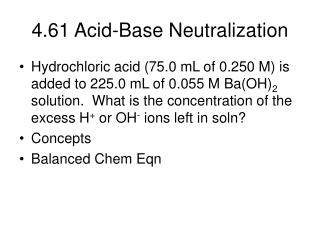DownloadDownload Presentation4.61 Acid-Base Neutralization

# 4.61 Acid-Base Neutralization

Télécharger la présentation## 4.61 Acid-Base Neutralization

- - - - - - - - - - - - - - - - - - - - - - - - - - - E N D - - - - - - - - - - - - - - - - - - - - - - - - - - -
##### Presentation Transcript

1. 4.61 Acid-Base Neutralization • Hydrochloric acid (75.0 mL of 0.250 M) is added to 225.0 mL of 0.055 M Ba(OH)2 solution. What is the concentration of the excess H+ or OH- ions left in soln? • Concepts • Balanced Chem Eqn

2. 4.61 Problem Solving Strategy • # mol acid available • # mol base available • # mol base needed to react with # mol acid available • Find LR and XS • # mol OH- available • # mol OH- that reacts • #mol OH- in excess • Total volume of soln • Molarity of OH-

3. 4.61: Find LR • Calculate # mol base needed to react with available acid) • (0.075 L x 0.250 M) x (1 mol Ba(OH)2 /2 mol HCl) = 0.009375 mol base needed • Calc # mol base available • (0.225 L x 0.055 M) = 0.012375 mol base available > 0.009375 mol based needed so base = XS and acid = LR

4. 4.61 Find #mol XS Reagent • Calculate # mol OH-available and #mol OH- that will react with LR = H+. • 0.012375 mol base x 2 mol OH-/ 1 mol base = 0.024750 mol OH-available • 0.009375 mol base x 2 mol OH-/ 1 mol base = 0.018750 mol OH-needed • (0.024750 mol OH- - 0.018750 mol OH-) = excess OH- = 0.00600 mol OH-

5. 4.61 Find Molarity of XS • Finally calculate molarity of excess OH- • Total volume = 0.300 L • M = #mol OH-/L soln = 0.00600mol/0.300L = 0.0200 M OH-

6. 4.66 Titration • Standardize a soln of NaOH with a known mass of KHP (monoprotic acid of molar mass = 204.22 g/mol). Ie determine [OH-] of base. • Mass of KHP = 0.1082 g • Volume of base = 34.67 mL • Concepts • Balanced Chem Eqn

7. 4.66 Problem Solving Strategy • # mol KHP • # mol base needed to neutralize available # mol KHP • Molarity of base

8. 4.66 Solution • #mol KHP = 0.1082g/204.22g/mol = 5.298E-4 mol KHP • Since neutralization rxn says one mol KHP or # mol H+ reacts with one mol base or one mol OH-, #mol base = 5.298E-4 mol base • #mol base = Mb x Vb • Mb = #mol base/Vb = 5.298E-4 mol/0.03467L = 0.01528 M